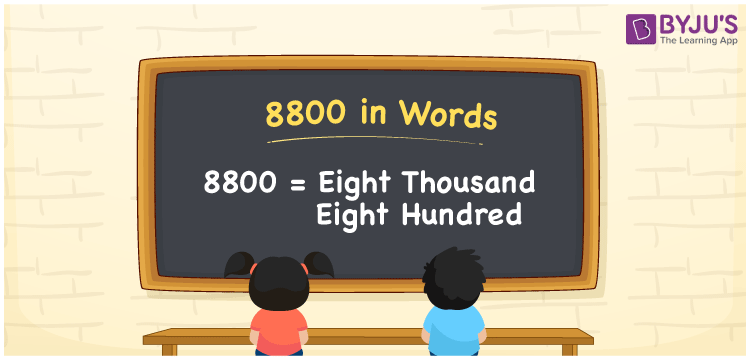# 8800 in words

8800 in words is written as Eight Thousand Eight Hundred. 8800 represents the count or value. The article on Counting Numbers can give you an idea about count or counting. The number 8800 is used in expressions that relate to money, distance, length and others. Let us consider an example for 8800. “15 years ago, the price of gold per 10 grams was Eight Thousand Eight Hundred Rupees.” Another example, “I had to delete around Eight Thousand Eight Hundred spam mails”.

 8800 in words Eight Thousand Eight Hundred Eight Thousand Eight Hundred in Numbers 8800

## 8800 in English Words## How to Write 8800 in Words?

We can convert 8800 to words using a place value chart. The number 8800 has 4 digits, so let’s make a chart that shows the place value up to 4 digits.

 Thousands Hundreds Tens Ones 8 8 0 0

Thus, we can write the expanded form as:

8 × Thousand + 8 × Hundred + 0 × Ten + 0 × One

= 8 × 1000 + 8 × 100 + 0 × 10 + 0 × 1

= 8800

= Eight Thousand Eight Hundred.

8800 is the natural number that is succeeded by 8799 and preceded by 8801.

8800 in words –Eight Thousand Eight Hundred.

Is 8800 an odd number? – No.

Is 8800 an even number? – Yes.

Is 8800 a perfect square number? – No.

Is 8800 a perfect cube number? – No.

Is 8800 a prime number? – No.

Is 8800 a composite number? – Yes.

## Solved Example

1. Write the number 8800 in expanded form

Solution: 8 × 1000 + 8 × 100 + 0 × 10 + 0 × 1.

We can write 8800 = 8000 + 800 + 0 + 0

= 8 × 1000 + 8 × 100 + 0 × 10 + 0 × 1.

## Frequently Asked Questions on 8800 in words

Q1

### How to write 8800 in words?

8800 in words is written as Eight Thousand Eight Hundred.
Q2

### State whether True or False. 8800 is divisible by 3?

False. 8800 is not divisible by 3.
Q3

### Is 8800 divisible by 10?

Yes. 8800 is divisible by 10. It is also divisible by 2, 4, 5, and 100.